CAT  >  Test: CAT Quantitative Aptitude- 1

# Test: CAT Quantitative Aptitude- 1

Test Description

## 22 Questions MCQ Test CAT Mock Test Series | Test: CAT Quantitative Aptitude- 1

Test: CAT Quantitative Aptitude- 1 for CAT 2022 is part of CAT Mock Test Series preparation. The Test: CAT Quantitative Aptitude- 1 questions and answers have been prepared according to the CAT exam syllabus.The Test: CAT Quantitative Aptitude- 1 MCQs are made for CAT 2022 Exam. Find important definitions, questions, notes, meanings, examples, exercises, MCQs and online tests for Test: CAT Quantitative Aptitude- 1 below.
Solutions of Test: CAT Quantitative Aptitude- 1 questions in English are available as part of our CAT Mock Test Series for CAT & Test: CAT Quantitative Aptitude- 1 solutions in Hindi for CAT Mock Test Series course. Download more important topics, notes, lectures and mock test series for CAT Exam by signing up for free. Attempt Test: CAT Quantitative Aptitude- 1 | 22 questions in 40 minutes | Mock test for CAT preparation | Free important questions MCQ to study CAT Mock Test Series for CAT Exam | Download free PDF with solutions
 1 Crore+ students have signed up on EduRev. Have you?
*Answer can only contain numeric values
Test: CAT Quantitative Aptitude- 1 - Question 1

### Some milk from a container, having 40 litres of milk, is drawn out and replaced with an equal amount of water. This process is repeated two more times and after that, only 20.48 litres of milk is left in the container. What is the amount of solution (in litres) removed in each iteration?

Detailed Solution for Test: CAT Quantitative Aptitude- 1 - Question 1

We can use the formula
Quantity left = Quantity present initially *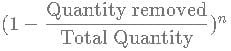Let the quantity removed in each iteration be x litres
Putting all values we get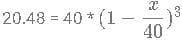Solving this equation we can get x = 8 litres
Hence, 8 is the correct answer.

*Answer can only contain numeric values
Test: CAT Quantitative Aptitude- 1 - Question 2

### Eight years ago, the ratio of ages of Akhil and Akash was 1:5. 12 years from now, the ratio changes to 7 : 15. Find the sum of the antecedent and the consequent of the ratio of their present ages, when the ratio is in its lowest form.

Detailed Solution for Test: CAT Quantitative Aptitude- 1 - Question 2

Let the present ages of Akhil and Akash be x and y respectively.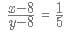⇒ 5x - 40 = y - 8
⇒ 5x - y = 32
⇒ y = 5x - 32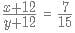⇒ 15x + 180 = 7y + 84
⇒ 15x + 180 = 7(5x - 32) + 84
⇒ 15x + 96 = 35x - 224
⇒ 20x = 320
⇒ x = 16
⇒ y = 48
x:y = 16:48 = 1:3
Sum of antecedent and consequent = 1 + 3 = 4

Test: CAT Quantitative Aptitude- 1 - Question 3

### Ratnesh bought a fridge and a television from big bazaar. He was promised 20% discount on the fridge and 25% discount on the television. However, a con salesman, interchanged the discount offered on both the items and processed the bill. If the mark price of the television is 4 times that of the fridge, then what is the ratio of the amount actually paid by Ratnesh to that he would have paid if he was not cheated?

Detailed Solution for Test: CAT Quantitative Aptitude- 1 - Question 3

Let Rs. 'x' be the mark price of the fridge. Then, the mark price of the television = Rs. 4x. Ratnesh was promised 20% discount on the fridge and 25% discount on the television. But the salesman interchanged the discounts on both the items. Therefore, Ramesh would have received 25% discount on the fridge whereas he would have received 20% discount on the television.
The actual amount which Ratnesh paid = 0.75 ∗ x + 0.80 ∗ 4x = 3.95x
The amount that he would have paid if he was not cheated = = 0.80*x + 0.75*4x = 3.80x
Hence, the ratio of the amount actually paid by Ratnesh to that he would have paid if he was not cheated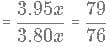Therefore, option B is the correct answer.

*Answer can only contain numeric values
Test: CAT Quantitative Aptitude- 1 - Question 4

Arun, Barun, Chandan and Diksha have books in the ratio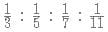respectively. What is the the minimum number of books they must be having altogether?
(Enter ‘0’ as the answer if the answer cannot be determined.)

Detailed Solution for Test: CAT Quantitative Aptitude- 1 - Question 4

The ratio of the books with them is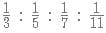LCM of 3, 5, 7 and 11 is 1155
So, they must be having books in the ratio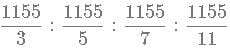which is equivalent to
385 : 231 : 165 : 105
Thus, the minimum number of books they must be having is
385 + 231 + 165 + 105 = 886
Hence, 886 is the correct answer.

Test: CAT Quantitative Aptitude- 1 - Question 5

Given that [(x-y)(1/4)] = 2 and [(3x−2y) 1/2] = 5. What is the minimum possible integral value of y? [x] is equal to greatest integer less than or equal to x.

Detailed Solution for Test: CAT Quantitative Aptitude- 1 - Question 5

Given in the question :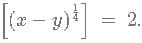The square bracket represent integer function.
Let us assume x - y = A.         (1)
The second equation mentioned was :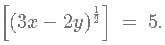Let 3x-2y = B                          (2)
In the question we were asked for the minimum possible value of y .
Multiplying (1) by and subtracting this from (2) we get :
3x-2y -(3x-3y) = y.
Hence y can be written as B - 3*A
In order to minimise y we must try to make the difference as low as possible and to do this we minimise the value of B to as low as possible and maximise A so the difference gets to its lowest value.
The minimum value of B = minimum value of square of 3x-2y
Since the integral part of the square root of B is 5. This must be in the range of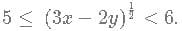The minimum value it can take is 5 and hence 3x-2y minimum value is 25.
The maximum value of A = maximum of x-y.
Since the integral part of fourth root of A is 2. This must be in the range of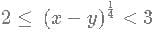The maximum value this cannot take is 81. But this takes values greater than 80 and less than 81 also.
So 3A can have a maximum value of 242.
The minimum value of B - 3A is 25 - 242 = -217

Test: CAT Quantitative Aptitude- 1 - Question 6

How many points in the region enclosed by x > 0, y < 0 and 7x - 9y < 63 have integral coordinates?

Detailed Solution for Test: CAT Quantitative Aptitude- 1 - Question 6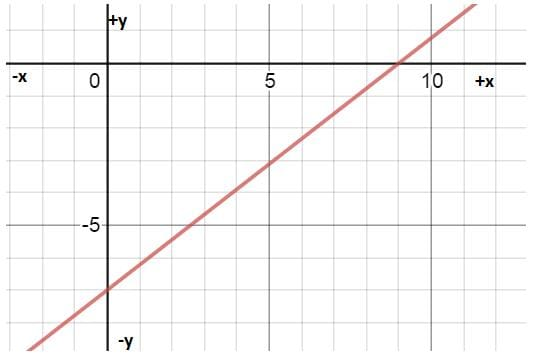The region enclosed by the lines is a triangle in the third quadrant formed by the points (0,-7), (0,0) and (9,0). The number of coordinates in the region with x coordinate are as follows
x=0 ⇒ 8 points,
x=1, 7points,
x=2, 6points,
x=3, 5points,
x=4, 4points,
x=5, 4points and so on.
Total no. of points =41

Test: CAT Quantitative Aptitude- 1 - Question 7

A circle with radius 6 cm is inscribed inside an equilateral triangle ABC. Three smaller circle are drawn touching the incircle and the sides of ABC as shown in the figure. Another triangle is formed by joining centres P,Q and R of these smaller circles. What is the perimeter of triangle PQR?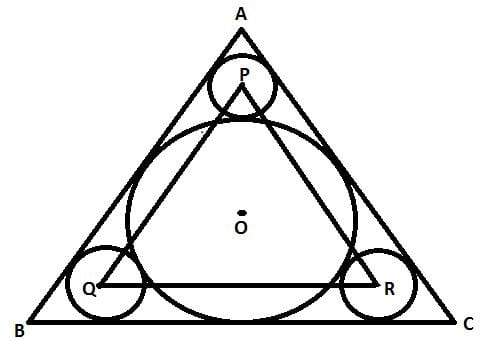Detailed Solution for Test: CAT Quantitative Aptitude- 1 - Question 7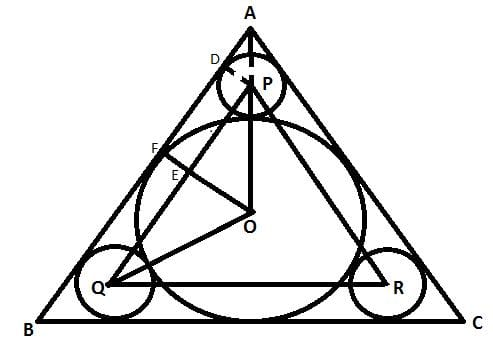Consider radius of each smaller circle be r and that of the larger circle be R = 6 cm.
Construct PD such that PD is perpendicular to AB.
In right triangle ADP, AP = DP/cos(APD) = DP/ cos(60) = 2DP = 2r
In right triangle OFA, OF/cos(AOF) = AO ⇒ R/(1/2) = AP+OP  ⇒ 2R=2r+r+R   ⇒ R = 3r
In right triangle OEP, EP=OPcos(EPO) = (R + r)((√3)/2 = (4R/3)((√3) = 2R/(√3)
PQ=2EP = 4R/√3)
Perimeter = 12R/(√3) = 24√3 cm (R = 6cm)

Test: CAT Quantitative Aptitude- 1 - Question 8

In a circle, two chords AB and CD intersect at a point E as shown in the figure. If AB = 6 cm and CD = 10 cm and ∠ OED = 30°, then find out the radius of the given circle.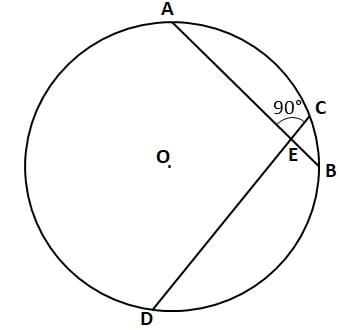Detailed Solution for Test: CAT Quantitative Aptitude- 1 - Question 8

Let us draw perpendicular from centre of the circle to AB and CD.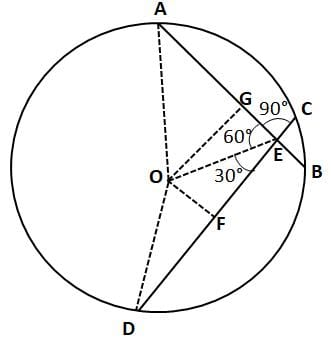We are given that ∠ OED = 30°, therefore ∠ AOE =90°-30° = 60°.
Let 'R' be the radius of the given circle. Then, in right-angle triangle ODF,
⇒ OF2 =OD2 −FD2
⇒ OF2 = R2 - 52
⇒ OF =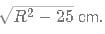In right-angle triangle OFE,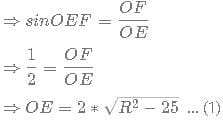Similarly, in right-angle triangle OGA,
⇒ OG2 = OA2 - AG2
⇒ OG =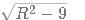In right-angle triangle OGE,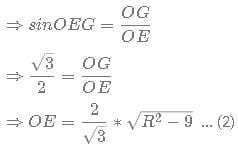By equating (1) and (2)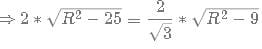⇒ 3*(R2-25)=R2-9
⇒ 2R2 =66
⇒ R = √33 cm
Hence, option C is the correct answer.

*Answer can only contain numeric values
Test: CAT Quantitative Aptitude- 1 - Question 9

How many scalene triangles with integral sides can be formed with a perimeter of 45 cm?

Detailed Solution for Test: CAT Quantitative Aptitude- 1 - Question 9

The number of triangles that can be formed for a given perimeter 'p' is given by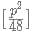when p is even and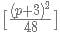when p is odd, where [] is the nearest integer function.
The number of scalene triangles that can be formed for a given perimeter 'p' is given by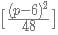when p is even and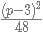if p is odd.
Number of scalene triangles that can be formed with a perimeter of 45 cm = [422 /48] = [36.75]= 37
Therefore, 37 is the correct answer.

Test: CAT Quantitative Aptitude- 1 - Question 10

AB and AC are tangents to the circle with centre O. If the radius of the circle is 5 and length of the tangent is 5√3, what is the area of the shaded region?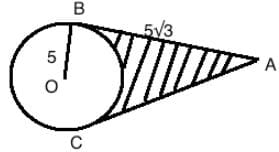Detailed Solution for Test: CAT Quantitative Aptitude- 1 - Question 10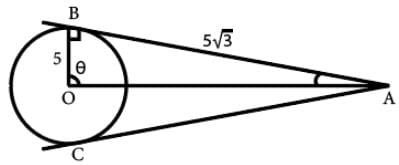In the triangle BOA, tan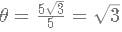Therefore, θ=60 degrees.
The shaded area = Area of two triangles - area of section of circle.
As the radius is perpendicular to a tangent, triangle OAB is a right angled triangle, with right angle at B, with area = 1/2 ∗ 5 ∗ 5√3​.
Hence area of both triangles= 2 ∗ 1/2 ∗ 5 ∗ 5√3​=25√3​.
The angle BOA =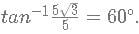Hence, angle BOC = 2*BOA = 120°
Hence the area of the section = area of circle/3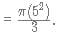Hence area of shaded portion=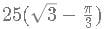Test: CAT Quantitative Aptitude- 1 - Question 11

In a quadrilateral ABCD, point E lies on side AB such that AE:EB = 2:3. If the point F, divides DE in 8:3 and DG = 20 cm, then find out the area of △BGC to that of △DGC.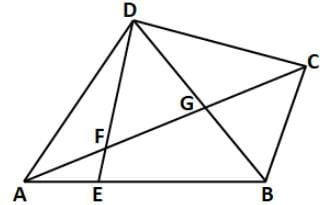Detailed Solution for Test: CAT Quantitative Aptitude- 1 - Question 11

Let us draw a line from point E that is parallel to AG and intersects DB at H.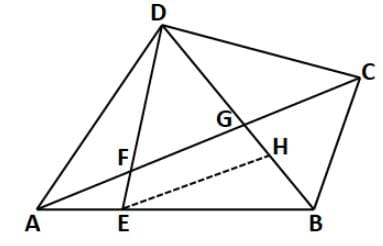The triangle DFG is similar to the triangle DEH. Hence,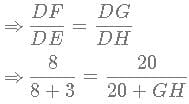⇒ GH = 7.5GH=7.5cm. ...(1)
Similarly, the triangle BEH is similar to the triangle BAG. Hence,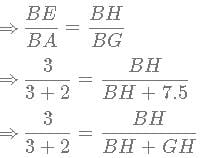⇒ BH = 11.25BH=11.25cm. ...(2)
From equation (1) and (2) we can say that BG = 7.5+11.25 = 18.75.
We can see that both the triangles △BGC and △DGC are of same height.
This is because their bases lie on the same line and have a common 3rd vertex. Hence, the perpendicular dropped from that vertex will be the same for both triangles.
Hence, the ratio of the area of the triangles will be same as the ratio of base lengths.Therefore,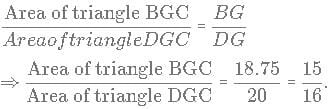Hence, option B is the correct answer.

Test: CAT Quantitative Aptitude- 1 - Question 12

The minimum value of the expression |x+3|+|x-3|+|x-6|+|x-5|+|x+5|

Detailed Solution for Test: CAT Quantitative Aptitude- 1 - Question 12

Let f(x) = |x+3|+|x-3|+|x-6|+|x-5|+|x+5|
f(x) is a linear equation in x at all times. It will be a union of straight lines with inflection points at -3, 3, 6, 5 and -5. Hence, the minimum value of the expression occurs at one of these inflection points. We will calculate the value of f(x) at each of these points and then find out the least possible value of f(x)
f(-3) = 25
f(3) = 19
f(6) = 24
f(5) = 21
f(-5) = 31
19 is the least value.

Test: CAT Quantitative Aptitude- 1 - Question 13

Find the number of non-negative integral points that satisfy 2x + y > 16 and x + 2y = 20.

Detailed Solution for Test: CAT Quantitative Aptitude- 1 - Question 13

Number of non-negative integral points on x + 2y = 20 ⇒ (20,0), (18,1),......,(2,9) and (0,10) ⇒ 11 points
Intersection point of 2x + y = 16 and x + 2y = 20 is (4,8)
So, all the points among the above 11 points that have x coefficient less than or equal to 4 are removed.
⇒ 3 points are removed.
Hence, required number of points = 11 - 3 = 8

Test: CAT Quantitative Aptitude- 1 - Question 14

Which condition will a,b,c satisfy if the set of equations 3x + 4y + z = a, 2x + 6y + 4z = b and x - y - 2z = c has atleast 1 solution ? Also a + b + c ≠ 0.

Detailed Solution for Test: CAT Quantitative Aptitude- 1 - Question 14

3x + 4y + z = a --> (1)
2x + 6y + 4z = b --> (2)
x - y - 2z = c --> (3)
Eliminating z from (1) and (2) , (1)x4 - (2) : 12x + 16y + 4z - (2x + 6y + 4z) = 4a - b
10x + 10 y = 4a - b
⇒ x + y = (4a - b)/10 - (4)
Eliminating z from (1) and (3), (1)x2 + 3 = 6x + 8y + 2z + (x - y - 2z) = 2a + c
⇒ x + y = (2a + c)/7 - (5)
Equating equations (4) and (5), we get,
(4a - b)/10 = (2a + c)/7
28a - 7b = 20a + 10c
Thus, 8a = 7b + 10c

Test: CAT Quantitative Aptitude- 1 - Question 15

Solve for x in the following equation:
log 10 (x−1) + log10 (2x-1)= 2 * log10 (x+1)2∗log 10 (x+1)

Detailed Solution for Test: CAT Quantitative Aptitude- 1 - Question 15

The equation can be written as follows: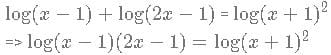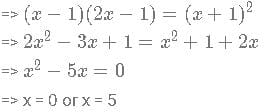For the logarithms in the question to be defined, x - 1 > 0 ⇒ x > 1
So, the only possible value of x = 5

Test: CAT Quantitative Aptitude- 1 - Question 16

If (|log (6x+4) (3x−2)|)=1. What is the number of possible values of x?

Detailed Solution for Test: CAT Quantitative Aptitude- 1 - Question 16

∣log (6x+4)(3x−2)∣=1
3x−2>0
x>2/3........(1)
log (6x+4) (3x−2)=±1
Case 1
log (6x+4) (3x−2)=1
∴ 6x+4 = 3x-2
∴ 6x+4=3x−2
x=-2
which is not possible according to the equation 1
Case 2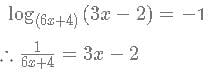(6x+4)(3x−2)=1
18x2 =9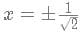1/√2 > 2/3
thus only one value of x i.e. 1/√2 can satisfy the equation.

Test: CAT Quantitative Aptitude- 1 - Question 17

Find the product of all the values of 3x+2 such that the values of xx satisfy the following equation: log3(18 - 3x) =3−x.

Detailed Solution for Test: CAT Quantitative Aptitude- 1 - Question 17

For the equation to be defined, 0 < 18 - 3x
So, the maximum value of x can be 2.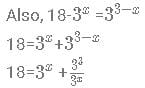Taking 3as t, then: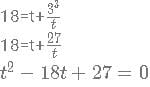Let us take the two roots to be A and B. A and B would be values of 3x  that satisfy the equation.
However, we need to find the product of 3x+2 that satisfy the equation. So, we must find the value of (A+2)*(B+2) = AB + 2*(A+B) + 4.
From the quadratic equation t^2-18t+27=0, we know that A+B = 18 and AB=27
So, the required product will be 27+36+4 = 67
So the answer is Option C

*Answer can only contain numeric values
Test: CAT Quantitative Aptitude- 1 - Question 18

What is the sum of all multiples of 3 less than 1000 which give an odd remainder when divided by 11 ?

Detailed Solution for Test: CAT Quantitative Aptitude- 1 - Question 18

The LCM of 3 and 11 is 33. Thus, the number of numbers we find in the first 33 numbers will be the number of numbers that are there in every consecutive set 33 numbers.
Multiples of 3 less than 33 that have odd remainders when divided by 11 : 3,9,12,18,27
In the next set of 33 numbers, the numbers that satisfy the conditions are : 36,42,45,51,60 ie 33+3,33+9,33+12,33+18,33+27 respectively.
Below 1000, there will be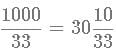which means there will be 30 such sets.
From 33x30 to 1000, ie from 990 to 1000, there are only 2 more numbers that satisfy the conditions ie 993 and 999.
To find sum of all the numbers, first we find the sum of the first series of numbers ie all numbers below 33 that satisfy the condition.
S1 =3+9+12+18+27=69
The sum of the second series of numbers S2 = (3+33)+(9+33)+(12+33)+(18+33)+(27+33) = 69 + (5 * 33) = 69+165
Similarly, the sum of the third series of numbers S3 =69+(165×2)
Thus, sum of the nth series of numbers Sn =69+[165×(n−1)]
Thus, total sum of the series till the 30th set of numbers S=(69×30)+[165×(1+2+3.....29)]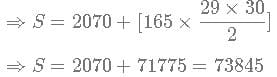We should remember that this series does not take into consideration the last 2 numbers.
Therefore the actual sum S' = 73845 + 993 + 999 = 75837

Test: CAT Quantitative Aptitude- 1 - Question 19

The difference between the roots of x22-2ax+3b=0 is same as the difference between the roots of x2 -2bx+3a=0, if a ≠ b then the sum a+b is

Detailed Solution for Test: CAT Quantitative Aptitude- 1 - Question 19

For a quadratic equation ax2 +bx+c=0, roots are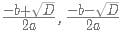where D = b2 + 4ac
Difference of roots =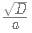Hence, 4a2-12b=4b2-12a
⇒ a2-3b+3a-b= 0
⇒ (a-b)(a+b)+3(a-b)=0
⇒ (a+b+3)(a-b)=0
Since a+b+3= 0, a+b =-3

*Answer can only contain numeric values
Test: CAT Quantitative Aptitude- 1 - Question 20

Let ABC be a right-angled triangle with BC as the hypotenuse. Lengths of AB and AC are 15 km and 20 krn, respectively. The minimum possible time, in minutes, required to reach the hypotenuse from A at a speed of 30 km per hour is

Detailed Solution for Test: CAT Quantitative Aptitude- 1 - Question 20

To find the time taken to reach from A to D we have to find length of AD.

⇒ Area of the ΔABC

⇒ (1/2) × BA × AC = (1/2) × BC × AD

⇒ (1/2) × 15 × 20 = (1/2) × 25 × AD

⇒ AD = 12 km

⇒ Required time = 12/30 = 2/5 hours = (2/5) × 60 = 24 minutes

∴ The required result will be 24 minutes.

*Answer can only contain numeric values
Test: CAT Quantitative Aptitude- 1 - Question 21

If a, b, c, and d are integers such that a + b + c + d = 30, then the minimum possible value of
(a - b)2 + (a - c)2 + (a - d)2 is

Detailed Solution for Test: CAT Quantitative Aptitude- 1 - Question 21

a + b + c + d = 30, a, b, c, d are integers.
(a – b)2 + (a – c)2 + (a – d)2 would have its minimum value when each bracket has the least possible value.
Let (a, b, c, d) = (8, 8, 7, 7)
The given expression would be 2.
It cannot have a smaller value

*Answer can only contain numeric values
Test: CAT Quantitative Aptitude- 1 - Question 22

DIRECTIONS for the question: Solve the following question and mark the best possible option.
A person can complete a job in 120 days. He works alone on Day 1. On Day 2, he is joined by another person who also can complete the job in exactly 120 days. On Day 3, they are joined by another person of equal efficiency. Like this, everyday a new person with the same efficiency joins the work. How many days are required to complete the job?

Detailed Solution for Test: CAT Quantitative Aptitude- 1 - Question 22

Time taken by a person to complete 1 job = 120
Work done by a person in 1 day = 1/120
Work done on 2nd day by 2 persons of same efficiency = 1/120 + 2/120 = 3/120
Work done on 3rd day by 3 persons . of same efficiency = 1/120 + 2/120 + 3/120 = 6/120
Work done on nth day by n persons of same efficiency = (1+2+3+4....... + n)/120
1 Job is completed on nth day. So the work done will be equal to 1:
(1+2+3+4....... + n)/120 = 1
n(n+1)/2 = 120 ( Sum of 1st n natural numbers = n(n+1)/2 )
Substituting the values from the options, we'll get n=15

## CAT Mock Test Series

2 videos|24 docs|89 tests
 Use Code STAYHOME200 and get INR 200 additional OFF Use Coupon Code
Information about Test: CAT Quantitative Aptitude- 1 Page
In this test you can find the Exam questions for Test: CAT Quantitative Aptitude- 1 solved & explained in the simplest way possible. Besides giving Questions and answers for Test: CAT Quantitative Aptitude- 1, EduRev gives you an ample number of Online tests for practice

## CAT Mock Test Series

2 videos|24 docs|89 tests

### How to Prepare for CAT

Read our guide to prepare for CAT which is created by Toppers & the best Teachers